Binary image convex hull

I've been intending to mention a new function bwconvhull that was introduced in the Image Processing Toolbox last spring in the R2011a release. Now that R2011b is out, I figure I better go ahead and do it!

bwconvhull computes the "convex hull of a binary image." Now I have to admit that this terminology is a little loose, so I'd better clarify. The convex hull of a set of 2-D points is the smallest convex polygon that contains the entire set. Here's an example from the MATLAB documentation for convhull:

xx = -1:.05:1; yy = abs(sqrt(xx));
[x,y] = pol2cart(xx,yy);
k = convhull(x,y);
plot(x(k),y(k),'r-',x,y,'b+')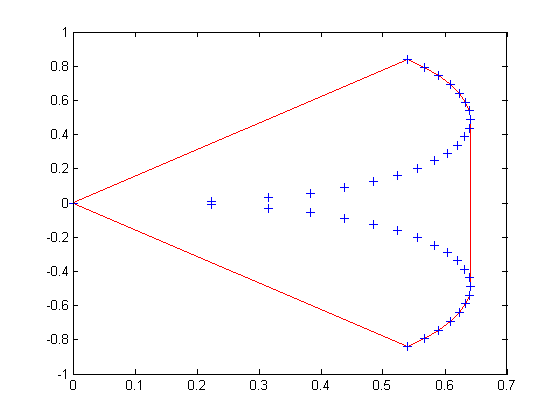The polygon in red is the convex hull of the set of points shown in blue. So convhull takes a set of points and returns a polygon, whereas bwconvhull takes a binary image and returns another binary image. What's up with that? It means simply that bwconvhull computes the convex hull of all the foreground pixels in the input image, and then it produces an output binary image with all the pixels inside the convex hull set to white. It's a little easier to show than to say, so here's what it looks like:

imshow(bw)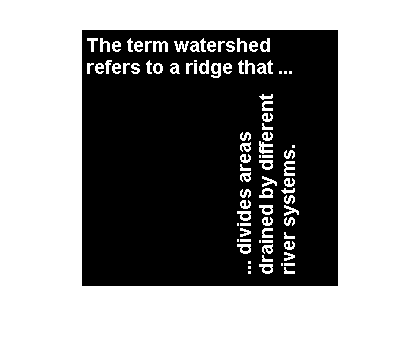bw2 = bwconvhull(bw);
imshow(bw2);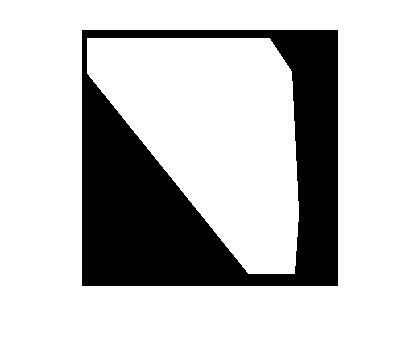Let's overlay the foreground pixel locations from the input image (using blue dots) and the convex hull computed by convhull (using a thick red line).

[y, x] = find(bw);
k = convhull(x, y);
hold on
plot(x, y, 'b.')
plot(x(k), y(k), 'r', 'LineWidth', 4)
hold off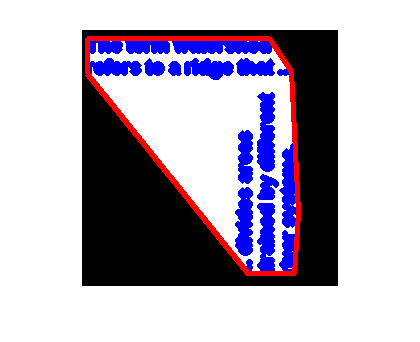An important variation supported by bwconvhull is to compute the convex hulls of the individual objects in the input image. Here's how you do that:

bw3 = bwconvhull(bw, 'objects');
imshow(bw3)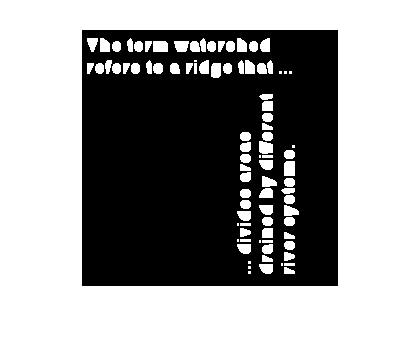Something called the convex deficiency is sometimes used in shape recognition applications. Loosely speaking, the convex deficiency of a shape is the convex hull of the shape minus the shape. Here's an example using the letter "T" from the text image above.

bwt = bw(7:24, 4:18);
imshow(bwt, 'InitialMagnification', 'fit')bwtc = bwconvhull(bw_t);
imshow(bwtc, 'InitialMagnification', 'fit')
title('Convex hull image')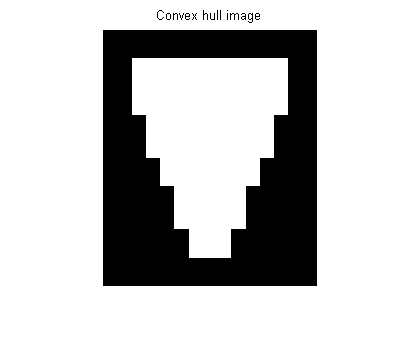bwtcd = bwtc & ~bwt;
imshow(bwtcd, 'InitialMagnification', 'fit')
title('Convex deficiency image')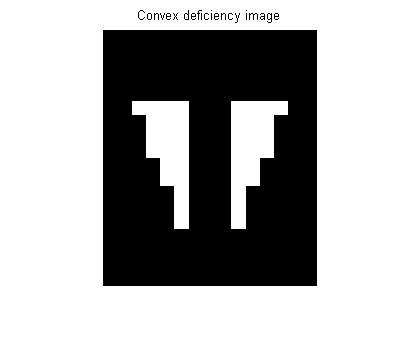The convex deficiency of the letter "T" has two connected components. This kind of measurement can be useful for recognizing shapes.

Published with MATLAB® 7.13

|

댓글

댓글을 남기려면 링크 를 클릭하여 MathWorks 계정에 로그인하거나 계정을 새로 만드십시오.